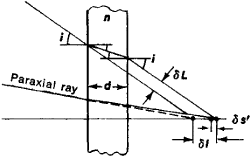# Plane-Parallel Plate

The following article is from The Great Soviet Encyclopedia (1979). It might be outdated or ideologically biased.

## Plane-Parallel Plate

a layer of a homogeneous medium that is bounded by parallel surfaces and is transparent for some range of wavelengths λ of optical radiation. The optical path of a light ray in a plane-parallel plate is nd, where d is the geometrical thickness of the plate, and n = n(λ) is the refractive index of the material of the plate. Suppose the angle of incidence i of a light ray on a plane-parallel plate is nonzero (Figure 1). When the ray passes through the plate, it preserves its direction as a result of being refracted twice at the surfaces of the plate but is displaced by the distance §L. The larger are i, d, and n, the greater is §L. When a plane-parallel plate is inserted into the path of a beam of rays forming an optical image of a point, the image is displaced by the distance δl. For a paraxial beam of rays whose axis is normal to the plate, δl = d(l — 1/n).Figure 1. Paths of light rays through a plane-parallel plate: (d) thickness of the plate, (n) refractive index of the material of the plate, (δl) plate-induced displacement of the image of a point along the axis perpendicular to the plate, (δL) transverse displacement of a ray incident on the plate at an oblique angle i; when i is large, the spherical aberration of the plate contributes to SI the additional displacement δs’ along the axis

Since optical objects that are not infinitely distant always emit beams of rays with i ≠ 0, a plane-parallel plate used as an optical element has aberrations, in particular, spherical aberration, chromatic aberration, and astigmatism. For sufficiently distant objects and sufficiently small d these aberrations are insignificant. When plane-parallel plates and reflecting prisms that are optically equivalent to such plates are incorporated in optical systems, these aberrations must be taken into account and corrected.

Plane-parallel plates are used as protective glass for windows and light filters (plane-parallel plates of colored materials). They are also used in goniometric devices (when the angular displacements of the image are small), in some interferometers, and as optical compensators.